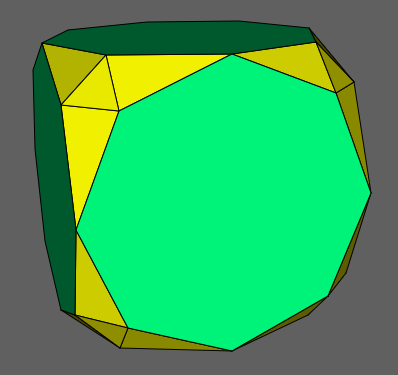Acronym retic (alt.: amtic) Name rectified/ambiated truncated cube© Circumradius 2+sqrt(2) = 3.414214

Rectification wrt. a non-regular polytope is meant to be the singular instance of truncations on all vertices at such a depth that the hyperplane intersections on the former edges will coincide (provided such a choice exists). Within the specific case of tic as a pre-image these intersection points might differ on its 2 edge types. Therefore tic cannot be rectified (within this stronger sense). Nonetheless the Conway operator of ambification (chosing the former edge centers generally) clearly is applicable. This would result in 2 different edge sizes in the outcome polyhedron. That one here is scaled such so that the shorter one becomes unity. Then the larger edge will have size k = sqrt[2+sqrt(2)] = 1.847759.

Incidence matrix according to Dynkin symbol

xo3oK4Ko&#zk   → height = 0
K = kk = 2+sqrt(2) = 3.414214 (pseudo)
k = x(8,2) = sqrt[2+sqrt(2)] = 1.847759
(tegum sum of (x,K)-sirco and K-co)

o.3o.4o.     | 24  * |  2  2 | 1  2 1
.o3.o4.o     |  * 12 |  0  4 | 0  2 2
-------------+-------+-------+-------
x. .. ..     |  2  0 | 24  * | 1  1 0
oo3oo4oo&#k  |  1  1 |  * 48 | 0  1 1
-------------+-------+-------+-------
x.3o. ..     |  3  0 |  3  0 | 8  * *  x-{3}
xo .. ..&#k  |  2  1 |  1  2 | * 24 *  xkk
.. oK4Ko&#zk |  4  4 |  0  8 | *  * 6  k-{8}